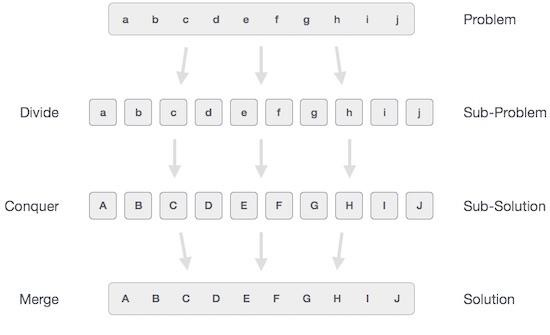# Python - Divide and Conquer

In divide and conquer approach, the problem in hand, is divided into smaller sub-problems and then each problem is solved independently. When we keep on dividing the subproblems into even smaller sub-problems, we may eventually reach a stage where no more division is possible. Those "atomic" smallest possible sub-problem (fractions) are solved. The solution of all sub-problems is finally merged in order to obtain the solution of an original problem.Broadly, we can understand divide-and-conquer approach in a three-step process.

## Divide/Break

This step involves breaking the problem into smaller sub-problems. Sub-problems should represent a part of the original problem. This step generally takes a recursive approach to divide the problem until no sub-problem is further divisible. At this stage, sub-problems become atomic in nature but still represent some part of the actual problem.

## Conquer/Solve

This step receives a lot of smaller sub-problems to be solved. Generally, at this level, the problems are considered 'solved' on their own.

## Merge/Combine

When the smaller sub-problems are solved, this stage recursively combines them until they formulate a solution of the original problem. This algorithmic approach works recursively and conquer &s; merge steps works so close that they appear as one.

### Examples

The following program is an example of divide-and-conquer programming approach where the binary search is implemented using python.

## Binary Search implementation

In binary search we take a sorted list of elements and start looking for an element at the middle of the list. If the search value matches with the middle value in the list we complete the search. Otherwise we eleminate half of the list of elements by choosing whether to procees with the right or left half of the list depending on the value of the item searched.

This is possible as the list is sorted and it is much quicker than linear search.Here we divide the given list and conquer by choosing the proper half of the list. We repeat this approcah till we find the element or conclude about it's absence in the list.

### Example

def bsearch(list, val):
list_size = len(list) - 1
idx0 = 0
idxn = list_size
# Find the middle most value
while idx0 <= idxn:
midval = (idx0 + idxn)// 2
if list[midval] == val:
return midval
# Compare the value the middle most value
if val > list[midval]:
idx0 = midval + 1
else:
idxn = midval - 1
if idx0 > idxn:
return None
# Initialize the sorted list
list = [2,7,19,34,53,72]

# Print the search result
print(bsearch(list,72))
print(bsearch(list,11))


### Output

When the above code is executed, it produces the following result −

5
None